Students can download Maths Chapter 6 Information Processing Ex 6.3 Questions and Answers, Notes, Samacheer Kalvi 6th Maths Guide Pdf helps you to revise the complete Tamilnadu State Board New Syllabus, helps students complete homework assignments and to score high marks in board exams.

## Tamilnadu Samacheer Kalvi 6th Maths Solutions Term 1 Chapter 6 Information Processing Ex 6.3

Question 1.
How many Triangles are there in each of the following figures?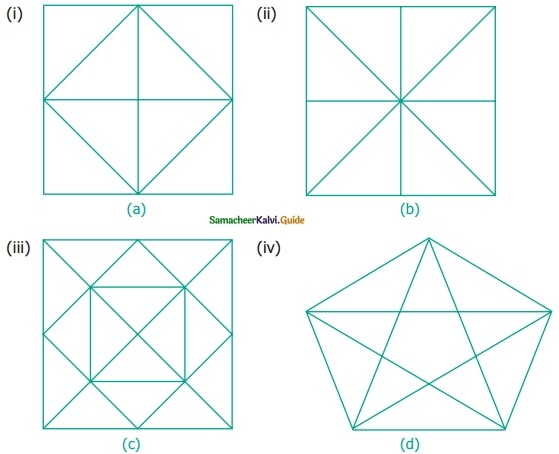Solution:
(i) 12 triangles
(ii) 16 triangles
(iii) 32 triangles
(iv) 35 trianglesQuestion 2.
Find the number of dots in the tenth figure of the following patterns.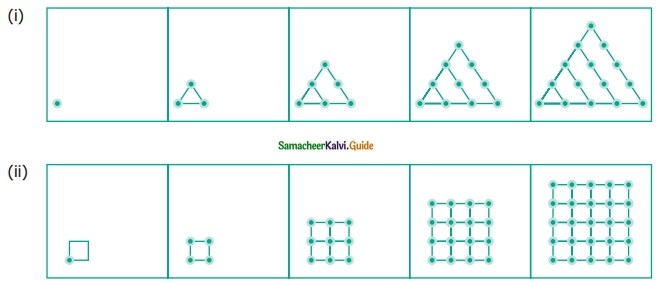Solution:
(i) 55
(ii) 100Question 3.(i) Draw the next pattern.
(ii) Prepare a table for the number of dots used for each pattern.
(iii) Explain the pattern.
(iv) Find the number of dots in the 25th pattern.
Solution: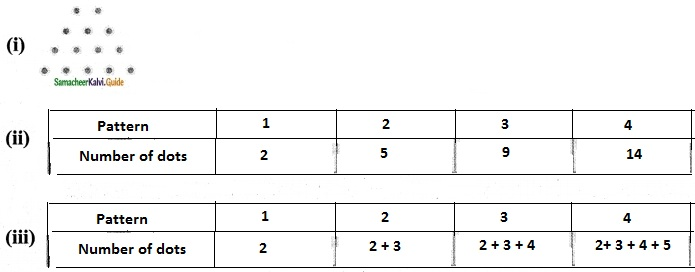(iv) 350Question 4.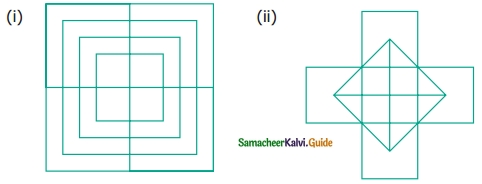Solution:
(i) 20 squares
(ii) 10 squaresQuestion 5.
How many circles are there in the following figure?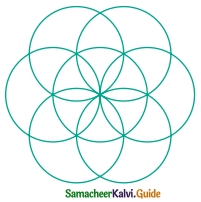Solution:
7 circlesQuestion 6.
Find the minimum number of straight lines used in forming the following figures.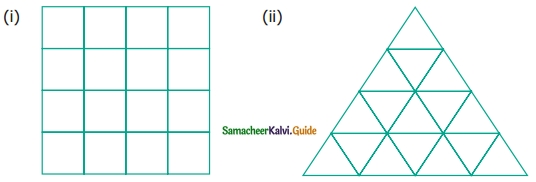Solution:
(i) 10
(ii) 12